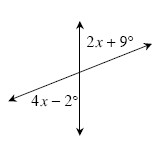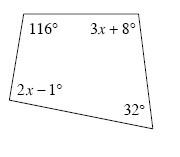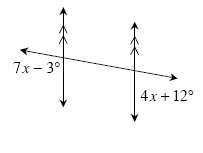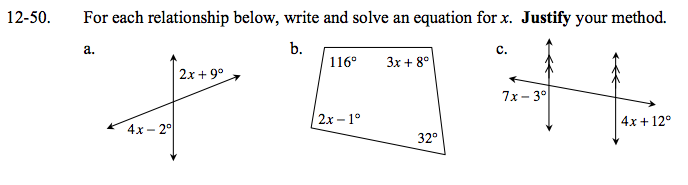### Home > GC > Chapter 12 > Lesson 12.1.5 > Problem12-50

12-50.
1. For each relationship below, write and solve an equation for x. Justify your method. Homework Help ✎

1.2.3.How are these angles related?

4x − 2° = 2x + 9°
11° = 2x
x = 55°

What is the sum of the angles of a parallelogram?

x = 41°

How are these angles related?